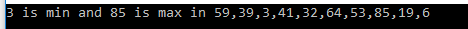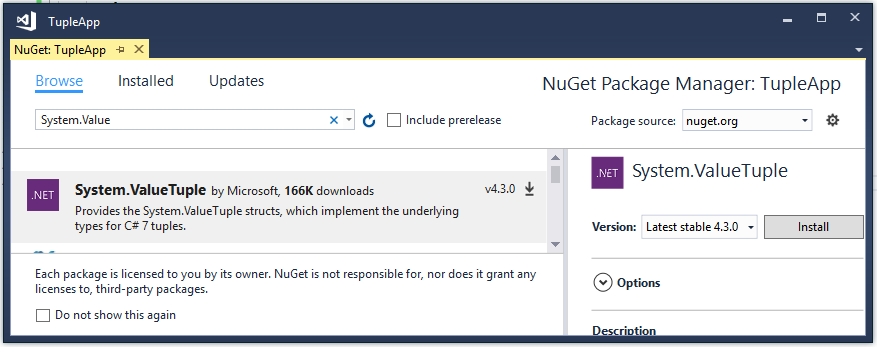# Tuple In C# 7

Intorduction

Tuple is an ordered sequence of heterogeneous objects. We may often write methods which return multiple values so we need to create a simple structure containing more than one data elements. To support these scenarios, tuples were added to C#. Tuples are lightweight data structures that contain multiple fields to represent the data members.

If a method returns multiple homogenous values, then it stores these values in the collection and returns that collection. But a method needs to return multiple heterogenous values, then C# provides some options such as Class/Struct, out parameter and Tuple.

Let’s create an example. We have a collection of integer numbers and we need to find out the minimum and maximum number from this collection. We need to create a method which returns both Minimum and Maximum values. To achieve this, we have three options which are Class/Struct, out parameter, and Tuple. So, let’s see each option one by one.

Using Out Parameter

As we create a method which finds the maximum and minimum number in the number series. This method has two values as a result of a maximum and minimum number. So, we create the method which returns a value and use out parameter as argument. The return value holds a maximum number from series while out parameter holds a minimum number from series.

Let’s create a class named Operation which holds a method to find a minimum and maximum number from number series. The following code snippet is for the same.

1. using System.Collections.Generic;
2.
3. namespace TupleApp
4. {
5.     class Operation
6.     {
7.         internal int FindMinMax(List<int> list, out int min)
8.         {
9.             int maximum = int.MinValue, minimum = int.MaxValue;
10.             list.ForEach(n =>
11.             {
12.                 minimum = n < minimum ? n : minimum;
13.                 maximum = n > maximum ? n : maximum;
14.             });
15.             min = minimum;
16.             return maximum;
17.         }
18.     }
19. }

As per the above code snippet, the method returns a value which holds an in variable named maximum. That value is a maximum number from series. This method takes out parameter as an argument named min which holds a minimum value from series.

Now, call this method from an executable program as per the following code snippet.

1. using System;
2. using System.Collections.Generic;
3. using System.Linq;
4. using static System.Console;
5.
6. namespace TupleApp
7. {
8.     class Program
9.     {
10.         static void Main(string[] args)
11.         {
12.             List<int> numbers = Enumerable.Range(1, 100).OrderBy(x => Guid.NewGuid()).Take(10).ToList();
13.             int minimum = 0, maximum = 0;
14.             Operation operation = new Operation();
15.             maximum = operation.FindMinMax(numbers, out minimum);
16.             Write(\$"{minimum} is min and {maximum} is max in {String.Join(",", numbers)}");
18.         }
19.     }
20. }

Now, run the application. The result shows as in the below figure.Figure 1: Output

It’s right approach but out parameter doesn’t work with async method.

Using Class/Struct

There is another option to get multiple values from a method - create a class or struct with that data structure. We perform the same operation here as well. We create a struct named CalculateData which has two properties to hold maximum and minimum number from a series. The following code snippet is used for the same struct.

1. namespace TupleApp
2. {
3.     struct CalculateData
4.     {
5.         public int Minimum { get; set; }
6.         public int Maximum { get; set; }
7.     }
8. }

Now, let’s create another class named Operation which holds a method to find a minimum and maximum number from number series. The following code snippet can be used for the same.

1. using System.Collections.Generic;
2.
3. namespace TupleApp
4. {
5.     class Operation
6.     {
7.         internal CalculateData FindMinMax(List<int> list)
8.         {
9.             int maximum = int.MinValue, minimum = int.MaxValue;
10.             list.ForEach(n =>
11.             {
12.                 minimum = n < minimum ? n : minimum;
13.                 maximum = n > maximum ? n : maximum;
14.             });
15.             CalculateData data = new CalculateData
16.             {
17.                 Minimum = minimum,
18.                 Maximum = maximum
19.             };
20.             return data;
21.         }
22.     }
23. }

As per the above code snippet, the method returns an object which has two properties. These properties hold maximum and minimum values from series.

Now, call this method from an executable program, as per the following code snippet.

1. using System;
2. using System.Collections.Generic;
3. using System.Linq;
4. using static System.Console;
5.
6. namespace TupleApp
7. {
8.     class Program
9.     {
10.         static void Main(string[] args)
11.         {
12.             List<int> numbers = Enumerable.Range(1, 100).OrderBy(x => Guid.NewGuid()).Take(10).ToList();
13.             Operation operation = new Operation();
14.             CalculateData data = operation.FindMinMax(numbers);
15.             Write(\$"{data.Minimum} is min and {data.Maximum} is max in {String.Join(",", numbers)}");
17.         }
18.     }
19. }

Now, run the application. The end result shows as in the below figure.Figure 2: Output

Using Tuple

The C# 7 introduced a new way to define Tuple. Tuple is another option to get multiple values from a method. It can hold multiple heterogeneous values. To use Tuple in the application, we need to install System.ValueTuple NuGet package.Figure 3 NuGet Package

We perform the same operation here as well. Let’s create a class named Operation which holds a method to find a minimum and maximum number from number series. The following code snippet is used for the same.

1. using System.Collections.Generic;
2.
3. namespace TupleApp
4. {
5.     class Operation
6.     {
7.         internal (intint) FindMinMax(List<int> list)
8.         {
9.             int maximum = int.MinValue, minimum = int.MaxValue;
10.             list.ForEach(n =>
11.             {
12.                 minimum = n < minimum ? n : minimum;
13.                 maximum = n > maximum ? n : maximum;
14.             });
15.             return (minimum, maximum);
16.         }
17.     }
18. }

As per the above code snippet, the method returns a tuple which has two items. These items hold maximum and minimum values from number series.

Now, call this method from an executable program as per the following code snippet.

1. using System;
2. using System.Collections.Generic;
3. using System.Linq;
4. using static System.Console;
5.
6. namespace TupleApp
7. {
8.     class Program
9.     {
10.         static void Main(string[] args)
11.         {
12.             List<int> numbers = Enumerable.Range(1, 100).OrderBy(x => Guid.NewGuid()).Take(10).ToList();
13.             Operation operation = new Operation();
14.             (intint) data = operation.FindMinMax(numbers);
15.             Write(\$"{data.Item1} is min and {data.Item2} is max from {String.Join(",", numbers)}");
17.         }
18.     }
19. }

As per the above code snippet, the method named FindMinMax calls and returns a tuple. When method directly returns more than one values into a Tuple type, it gives them some default name as per their order so as to call them easily. This tuple has two items so these items call Item1 and Item2. Item1 represents first value while Item2 represent second value. Tuple items are used in the same sequence which they are created in.

Now, run the application. The final result shows as in the below figure.Figure 4 Output

As Item1 and Item2 don’t represent actual names of fields, so we can define custom names of them. Tuple type variables can have custom names instead of Item1 or Item2.

Let’s update the existing method named FindMinMax in the Operation class. We assign custom names to those tuple fields. The following code snippet is used for the same.

1. using System.Collections.Generic;
2.
3. namespace TupleApp
4. {
5.     class Operation
6.     {
7.         internal (int Minimum, int Maximum) FindMinMax(List<int> list)
8.         {
9.             int maximum = int.MinValue, minimum = int.MaxValue;
10.             list.ForEach(n =>
11.             {
12.                 minimum = n < minimum ? n : minimum;
13.                 maximum = n > maximum ? n : maximum;
14.             });
15.             return (minimum, maximum);
16.         }
17.     }
18. }

Here, the first field of the tuple has name Minimum while another field has name Maximum.

Now, call this method from an executable program as per the following code snippet.

1. using System;
2. using System.Collections.Generic;
3. using System.Linq;
4. using static System.Console;
5.
6. namespace TupleApp
7. {
8.     class Program
9.     {
10.         static void Main(string[] args)
11.         {
12.             List<int> numbers = Enumerable.Range(1, 100).OrderBy(x => Guid.NewGuid()).Take(10).ToList();
13.             Operation operation = new Operation();
14.             var data = operation.FindMinMax(numbers);
15.             Write(\$"{data.Minimum} is min and {data.Maximum} is max from {String.Join(",", numbers)}");
17.         }
18.     }
19. }

The method named FindMinMax calls and returns a tuple. This tuple has two items that call Minimum and Maximum values as per the sequence of tuple items.

Now, run the application and see the result.Figure 5 Output

Conclusion

Tuple is an ordered sequence of heterogeneous objects. It is used when a method is required to return more than one values.Tuple instance has a fixed number of items. Tuples have a limit of 8 items. If we want to create a Tuple with more items, we have to create nested Tuples. The eighth item of a Tuple should necessarily be another Tuple.

Next » Binary Literal, Digit Separator, Local Functions